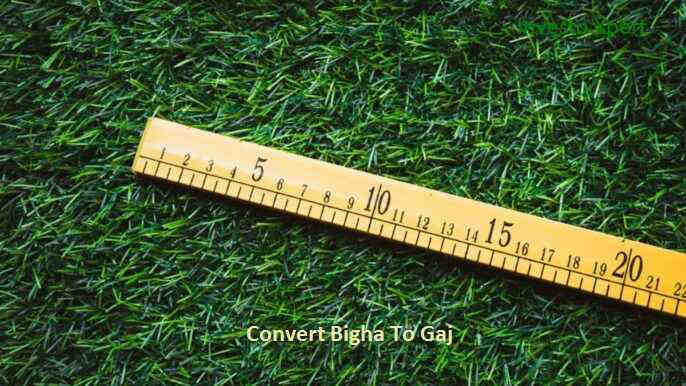How Many 1 Bigha in Gaj, Calculate 1 Bigha to Gaj?

# How Many 1 Bigha in Gaj, Calculate 1 Bigha to Gaj?

By Investoxpert | 03 Aug 2021 | Real EstateHow Many 1 Bigha in Gaj, Calculate 1 Bigha to Gaj, In different states of India, the unit of measurement for land is different. And, for that specific region uses a particular unit only. So, in this blog we will understand the various terms used for measurement.

What Is Bigha?

Bigha is a commonly used term that is useful in measuring land areas in India Nepal, Bangladesh, and Fiji. It is a familiar word though it does not have any type of standard size, yet, it is said to be used in most states of India, namely Haryana, Uttarakhand, Himachal Pradesh, Haryana, Madhya Pradesh, Punjab, Jharkhand, Bihar, Uttar Pradesh, Assam, West Bengal, Rajasthan and in Gujarat.

### Read also:Top 10 Best Luxury Apartments in Gurgaon

Even, the southern states present within the Indian geography make use of a different term rather than Bigha. As the size of Bigha differs from place to place, so beforehand you need to check with the same to calculate or convert 1 Bigha in Gaj or other units.

Bigha in the English language means “a land area unit”.

### Read Also: Top 10 Best Luxury Apartments in Noida

What Is Gaj?

Gaj is a Hindi term and in simple layman’s language it is used to as a measuring unit for a piece of land. It comes to use widely in the real estate sector that helps in measuring land and building structures as well.

During the early British ruling period, people used to follow Gaj to wisely measure both the length and breadth of an area. Gaj is called a GAJ in the English language and one Gaj is equal to nine square feet, which means one hundred Gaj is equal to nine hundred square feet.

So, to simply calculating you need to multiply the measured Gaj unit with the decimal nine to find out the exact square feet a particular area holds onto.

Also, 100 Gaj in Bishwa is almost equal to 0.66666666666667.

### Read Also:- Top 10 Best Residential Projects in Mumbai

How To Calculate Gaj In Bigha?

Mathematically calculating using a simple formula:

If you are quite good at mathematics and quite familiar in solving equations, then you can follow up on the conversion formula as mentioned below to calculate and convert Bigha Into Gaj.

1 Bigha= 1600 Gaj when converting it for land measurement purposes.

1 acre = 4840 Gaj, which means two acres is equal to 9680. Make use of the decimal 4840 and multiply it with the attained acre value to convert acre to Gaj at ease.

1 Biswa in Gaj is simply equal to 60.500504, so just multiply X Bishwa into 60.500504 to convert it into Gaj.

Accessing Online Land Measurement Calculators

There are many online calculators available that can be used to make use of online land measurement that are solely created to meet up with this kind of conversion purpose.

Such calculators are derived using the exact formula as mentioned earlier to find out the real value that you are looking forward to calculating in the first place.

### Read Also:TVS Emerald Singasandra Bangalore

1 Bigha To Gaj In Uttar Pradesh:

Bigha gets sub-divided and called as Biswa (Bisa) or Katta(Katha) in Uttar Pradesh. Usually,1 Bigha is equal to five or two hundred Biswa, and it does differ based upon the location in which you are about to calculate a piece of land in the state of Uttar Pradesh.

1 Bigha=20 Biswas

1 Bigha=3000.025000 Gaj

1 Bigha= 27000 Square feet.

1 Bigha To Gaj In Rajasthan:

Unlike the state of Uttar Pradesh, Rajasthan does have its sub-divided Bigha units in the name of Pucca and Kaccha.

In Rajasthan,

One Pucca Bigha = 27,255 square feet or Gaj.

One Kaccha Bigha = 1,618.7 square meters or 17,424 square feet.

1 Bigha sq feet in Rajasthan (around Jaipur) is calculated as 165 feet or 2532 square meters.

West Bengal:

1 Bigha Gaj is calculated as 1333 m2 in terms of the metric unit system. During the British colonial rule, one Bighawas equivalent to 0.3306 acres.

1 Bigha= 1615.509238 Gaj

2 Bigha= 3231.018476 Gaj

3 Bigha= 4846.527714 Gaj

4 Bigha= 6462.036952 Gaj

5 Bigha= 8077.546190 Gaj

### Read Also: M3M Sector 128 Noida

One Katha or Bigha is equivalent to six hundred Gaj or square feet.

1 Bigha= 1346.257698 Gaj

2 Bigha= 2692.515397 Gaj

3 Bigha= 4038.773095 Gaj

4 Bigha= 5385.030793 Gaj

5 Bigha= 6731.288492 Gaj

Haryana and Punjab:

One acre is equal to 4.8 Bigha.

1 Bigha= 1210.010083 Gaj

2 Bigha= 2420.020167 Gaj

3 Bigha= 3630.030250 Gaj

4 Bigha= 4840.040334 Gaj

5 Bigha= 6050.050417 Gaj

Five Bigha is equal to 1 acre or 0.0809 hectares.

1 Bigha= 977.383089 Gaj

2 Bigha= 1954.766178 Gaj

3 Bigha= 2932.149267 Gaj

4 Bigha= 3909.532356 Gaj

5 Bigha= 4886.915445 Gaj

### Read Also: M3M Sector 111 Gurgaon

Bihar:

In Bihar, one bigha is equal to 20 Katha.

1 Bigha= 3053.761212 Gaj.

2 Bigha= 6107.522425 Gaj.

3 Bigha= 9161.283637 Gaj.

4 Bigha= 12215.044850 Gaj.

5 Bigha= 15268.806062 Gaj.

Bigha is also called Lagga in the local term and people use their hand lengths in ancient periods to measure a particular area.

Assam:

1 Bigha is equal to 1,340 m2 or 14,400 square feet.

In Assam, the Bigha gets sub-divided into Kathas.

1 Katha In Gaj has a measurement value of 2,880 square feet of Gaj.

The local people make use of the term ‘Pura’ to denote 4 Bighas.

Conversion Table Bigha To Gaj In Assam

1 Bigha=1600 Gaj

2 Bigha=3200 Gaj

3 Bigha=4800 Gaj

4 Bigha=6400 Gaj

5 Bigha=8000 Gaj

6 Bigha=9600 Gaj

7 Bigha=11200 Gaj

8 Bigha=12800 Gaj

9 Bigha=14400 Gaj

10 Bigha=16000 Gaj

Thus, this will surely help you in easy conversion of land measurements. Hope, you find this blog useful!!!

#1bighaingaj
#1bighatogaj
#gajin1bigha